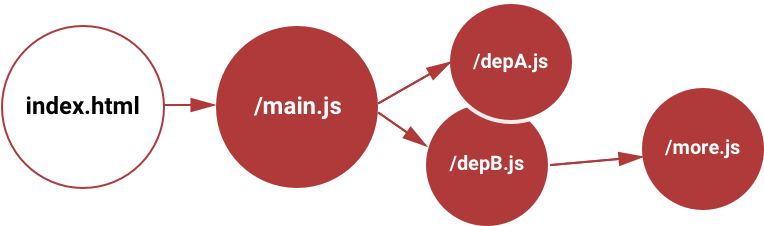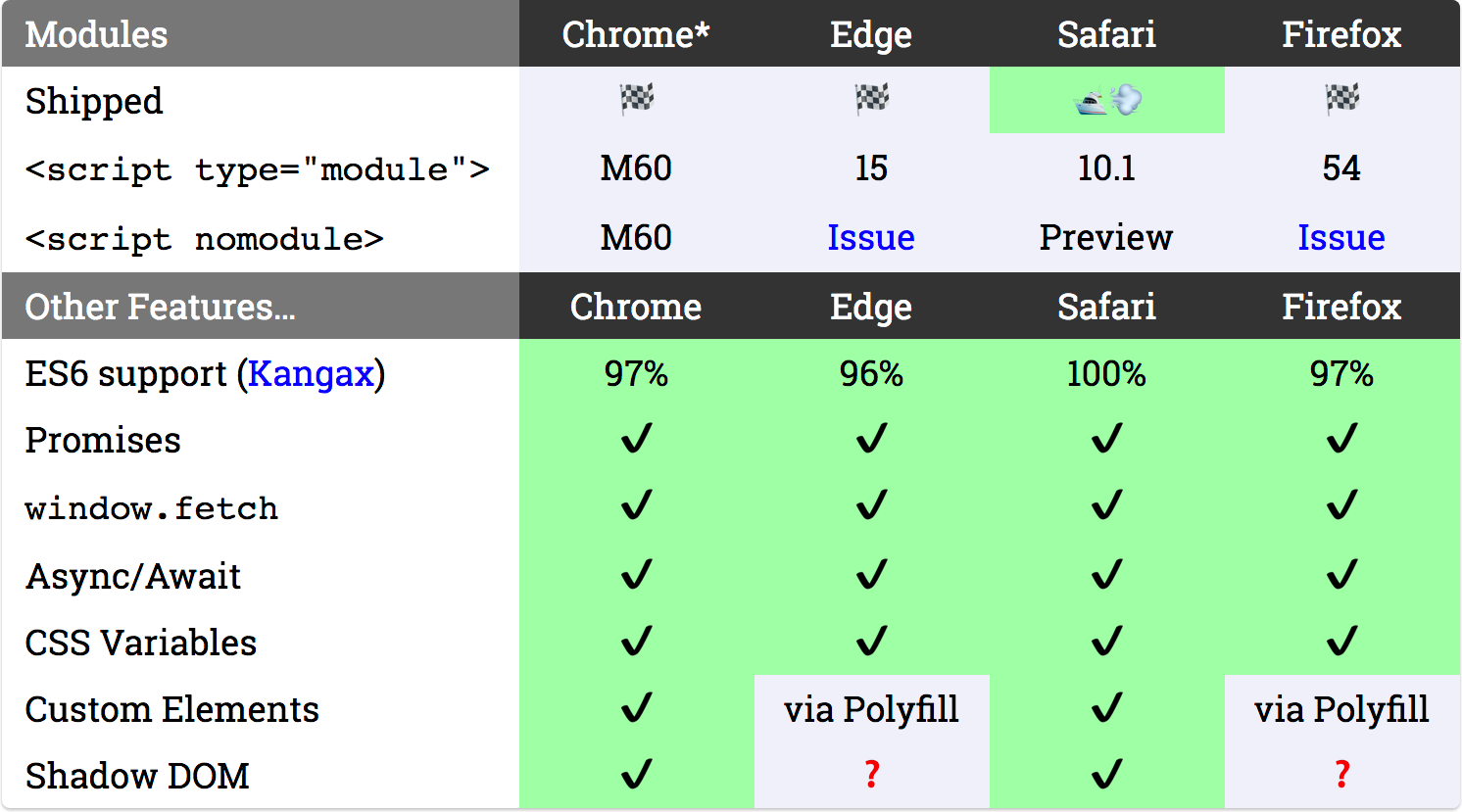# Introduction to ES6 modules

Subscribe to my newsletter and never miss my upcoming articles

An essential aspect of software engineering is efficiency. Every successful app needs a solid architectural structure that allows it to scale at all levels without breaking. In respect to this, several techniques and patterns are engaged to ensure code efficiency.

In this article, I would introduce you to ES6 modules, what they are, how to use them and why you should care {0[]0}

### What exactly is a module?

In JavaScript, the word “modules” refers to small units of independent, reusable code. They are the foundation of many JavaScript design patterns and are critically necessary when building any substantial JavaScript-based application.

In simpler terms, modules help you to write code in your module and expose only those parts of the code that should be accessed by other parts of your code.

JavaScript has had modules for a long time. However, they were implemented via libraries, not built into the language. ES6 is the first time that JavaScript has built-in modules.

• Each module is a piece of code that is executed once a JavaScript file is loaded.
• In that code, there may be declarations (variables, functions, classes e.t.c).
• By default, every declaration in that file stay local to that module and cannot be accessed in other modules unless the module file exports them.

### What are ES6 Modules?

Before now, it was impossible to directly reference or include one JavaScript file in another, as such, developers therefore resorted to alternative options like multiple HTML script tags

``````<script src="app.js"></script><script src="search.js"></script><script src="user.js"></script><script>console.log('inline code');</script>
``````

This is a bad practice as each script initiates a new HTTP request, which affects page performance and disrupts further processing while it runs.

Let's say we have a file, `app.js` and included in this file is a function which checks every limit in a number and returns if it’s EVEN or ODD.

``````function showNumbers(limit) {
(let i = 0; i <= limit; i++) {
const message = (i % 2 === 0) ? 'EVEN' : 'ODD';
console.log(i, message);
}
}
``````

Now this function is only available within `app.js` . Wherever you need this function, you have to either rewrite the function or attach the script again.

This is where ES6 Modules come in :)

With ES6 modules, you can concatenate all scripts in one main script by marking some of them as exports, then other modules can import them.How ES6 modules work.

Before ES2015 release, there were at least 3 major modules competing standards:

• Asynchronous Module Definition (AMD)
• RequireJS Modules
• CommonJS Modules

A single, native module standard was therefore proposed in ES6 (ES2015).

ES6 modules is a very powerful concept, although support is not available everywhere yet, a common way of using it is to transpile into ES5. You can use Grunt, Gulp, Webpack, Babel or some other transpiler to compile the modules during a build process.

Transpilers, or source-to-source compilers, are tools that read source code written in one programming language, and produce the equivalent code in another language. (Wikipedia)

Browsers with ES6 modules support, May 2017. [_Source_]### What you should know!

• Everything inside an ES6 module is private by default, and runs in strict mode (there’s no need for `'use strict'`).
• Public variables, functions and classes are exposed using `export`.
• Exposed modules are called into other modules using `import`
• Modules must be included in your HTML with `type="module"`, which can be an inline or external script tag.
``````</script>
<script type="module">
// or an inline script
</script>
``````
• Modules are deferred, and only run after a document is loaded

### How to use ES6 modules

For this article, let's create three files, (`app.js` ,`math.js` and `max.js` )

In `math.js` , let's write a basic arithmetic algorithm

``````let sumAll = (a, b) => {return a + b;}
``````
``````let subtractAll = (a, b) => {return a - b;}
``````
``````let divideAll = (a, b) => {return a / b;}
``````
``````let multiplyAll = (a, b) => {return a * b;}
``````
``````let findModulus = (a, b) => {return a % b;}
``````

Now to make this module accessible to other parts of our code, we need to `**export**` them. There are several ways of doing this;

• You can export each module individually:
``````export let sumAll = (a, b) => {return a + b;}
``````
``````export let subtractAll = (a, b) => {return a - b;}
``````
``````export let divideAll = (a, b) => {return a / b;}
``````
``````export let multiplyAll = (a, b) => {return a * b;}
``````
``````export let findModulus = (a, b) => {return a % b;}
``````
• A better way is to use a single export statement.
``````let sumAll = (a, b) => {return a + b;}
``````
``````let subtractAll = (a, b) => {return a - b;}
``````
``````let divideAll = (a, b) => {return a / b;}
``````
``````let multiplyAll = (a, b) => {return a * b;}
``````
``````let findModulus = (a, b) => {return a % b;}
``````
``````export {sumAll, subtractAll, divideAll, multiplyAll, findModulus};
``````
• You can also export default values
``````export default {sumAll, subtractAll, multiplyAll, divideAll};
``````

Note: You only export modules you want to use in other parts of your code, its not mandatory for you to export every module in this script

Now to use our exported modules, we need to import them in `app.js` There are several ways of doing this also;

• You can import individually
``````import {sumAll} from './math.js';
``````

Let's test our import

``````console.log(sumAll(9, 8)); //17
``````
• You can import multiple modules at once:
``````import {sumAll, subtractAll, divideAll} _from_ './math.js';
``````
• You can import modules as aliases

In some computer operating systems and programming languages, an alias is an alternative and usually easier-to-understand or more significant name for a defined data object. More details here

``````import * as math from './math.js';
``````

Here we have imported all the modules in `math.js` and assigned them to the alias `math` . To use this imported module we do this:

``````console.log(math.sumAll(50, 10)); // 60
``````
``````console.log(math.subtractAll(50, 10)); // 40
``````
``````console.log(math.multiplyAll(50, 10)); // 500
``````
``````console.log(math.divideAll(50, 10)); // 5
``````
``````console.log(math.findModulus(50, 15)); // 5
``````
• You can also import default values
``````import math from './math.js';
console.log(math.sumAll(5, 2)); // 7
``````

Here we have imported `sumAll()` in `math.js` and assigned it to the alias `math` . There is no need to add `* as` here.

If you don’t export as `default` and you import using this method, you will get this error:

``````**Uncaught SyntaxError: The requested module './math.js' does not provide an export named 'default'**
``````

To use this method, you must export `sumAll()` as `default`

``````export default {sumAll};
``````
• You can also use an absolute path for the module import, to reference modules defined on another domain:
``````import {sumAll} from 'https://bolaji-module.glitch.me/sumAll.js';
console.log(sumAll(50, 10)); // 60
``````

Note: Modules are fetched using CORS. This means that if you reference scripts from other domains, they must have a valid CORS header that allows cross-site loading.

Modules are imported with either absolute or Relative references and must start with either “/”, “./”, or “../”.

``````import {sumAll} from 'math.js'; ❌
import {sumAll} from 'lib/max.js'; ❌
``````
``````import {sumAll} from './math.js'; ✔️
import {sumAll} from '../max.js'; ✔️
`import {`sumAll} `from '`https://bolaji-module.glitch.me/sumAll.js'; ✔️
``````
• You can import several modules from different files, in our `max.js` add this:
``````let max = (a, b) => {
_return_ (a > b) ? a : b;
}
export {max};
``````

Now you can import `max.js` and `math.js` together in `app.js`

``````import * as math from './math.js';
import {max} from './max.js';
``````
``````console.log(max(50, 10)); // 50
``````
``````console.log(math.subtractAll(50, 10)); // 40
``````
``````console.log(math.multiplyAll(50, 10)); // 500
``````
``````console.log(math.divideAll(50, 10)); // 5
``````

You can import multiple files but we must also remember that having more than a few modules are going to reduce our app’s performance, only use modules when needed.

### Some Advantages of using modules

• Code can be split into smaller files of self-contained functionality.
• Multiple scripts can be concatenated thereby increasing performance.
• Debugging becomes easier.
• Any code referencing a module understands it is a dependency. If the module file is changed or moved, the problem is immediately obvious.
• Modules (usually) helps eradicate naming conflicts. Function `sumAll()` in module1 cannot clash with Function `sumAll()` in module2. Aliases are very useful here, it becomes `module1.sumAll()` and `module2.sumAll()`.
• ES6 Modules are always executed in `strict mode`, so there is no need for `‘use strict’` .

### CONCLUSION

ES6 Modules are one of the biggest features introduced in modern browsers. Modern JavaScript frameworks like Vue JS and React JS use this feature.

You should also know that ES6 modules are not supported in all browsers. For production applications, transpilers like Webpack and Babel are used to convert our code from ES6 to ES5 to ensure cross-browser compatibility

In respect to this article, I would be writing two more articles tomorrow to show you how to useBabel as your transpiler (The article will cover an introduction guide to installing and setting up Babel) and how to efficiently work with `node_modules` in development and production.

Subscribe to my newsletter here and get notified when it drops!

So this it, while you await the ‘Babel transpiler article’ practice all you learnt from this article, use it in different projects and try all methods explained to get a better grasp of ES6 modules.

#### UPDATE

Here’s the article on Introduction to Babel and JavaScript Bundlers

Two things you need to do

Use a Live server to run your HTML files.

Don't forget to add `type="module"` when adding your main script